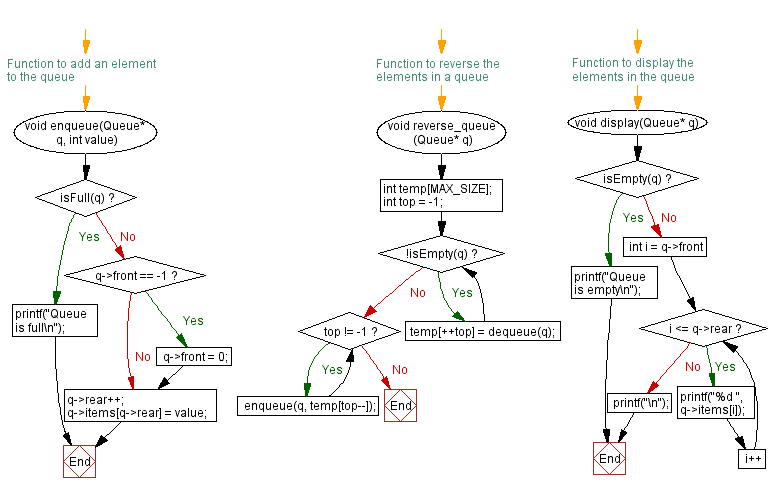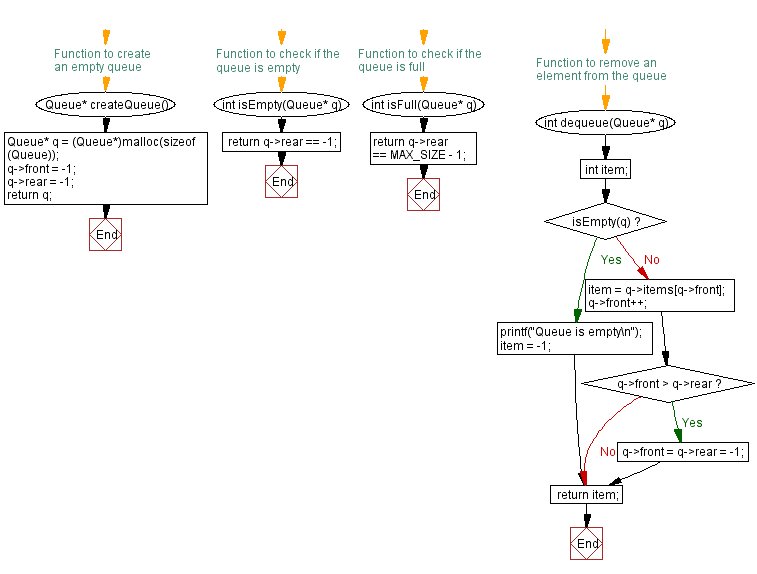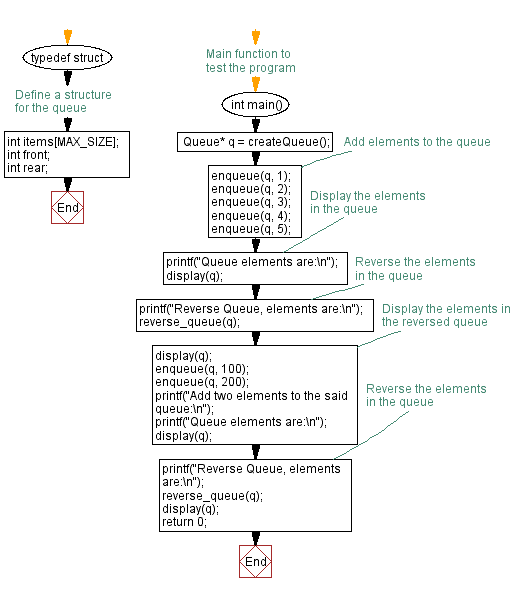﻿ C - Reverse the elements of a queue

# C Exercises: Reverse the elements of a queue

## C Queue: Exercise-6 with Solution

Write a C program to reverse the elements of a queue.

Sample Solution:

C Code:

``````#include <stdio.h>
#include <stdlib.h>

#define MAX_SIZE 100
// Define a structure for the queue
typedef struct {
int items[MAX_SIZE];
int front;
int rear;
} Queue;

// Function to create an empty queue
Queue* createQueue() {
Queue* q = (Queue*)malloc(sizeof(Queue));
q->front = -1;
q->rear = -1;
return q;
}

// Function to check if the queue is empty
int isEmpty(Queue* q) {
return q->rear == -1;
}

// Function to check if the queue is full
int isFull(Queue* q) {
return q->rear == MAX_SIZE - 1;
}
// Function to add an element to the queue
void enqueue(Queue* q, int value) {
if (isFull(q)) {
printf("Queue is full\n");
} else {
if (q->front == -1) {
q->front = 0;
}
q->rear++;
q->items[q->rear] = value;
}
}

// Function to remove an element from the queue
int dequeue(Queue* q) {
int item;
if (isEmpty(q)) {
printf("Queue is empty\n");
item = -1;
} else {
item = q->items[q->front];
q->front++;
if (q->front > q->rear) {
q->front = q->rear = -1;
}
}
return item;
}

// Function to reverse the elements in a queue
void reverse_queue(Queue* q) {
int temp[MAX_SIZE];
int top = -1;
while (!isEmpty(q)) {
temp[++top] = dequeue(q);
}

while (top != -1) {
enqueue(q, temp[top--]);
}
}
// Function to display the elements in the queue
void display(Queue* q) {
if (isEmpty(q)) {
printf("Queue is empty\n");
} else {
for (int i = q->front; i <= q->rear; i++) {
printf("%d ", q->items[i]);
}
printf("\n");
}
}

// Main function to test the program
int main() {
Queue* q = createQueue();

// Add elements to the queue
enqueue(q, 1);
enqueue(q, 2);
enqueue(q, 3);
enqueue(q, 4);
enqueue(q, 5);
// Display the elements in the queue
printf("Queue elements are:\n");
display(q);
// Reverse the elements in the queue
printf("Reverse Queue, elements are:\n");
reverse_queue(q);
// Display the elements in the reversed queue
display(q);
enqueue(q, 100);
enqueue(q, 200);
printf("Add two elements to the said queue:\n");
printf("Queue elements are:\n");
display(q);
// Reverse the elements in the queue
printf("Reverse Queue, elements are:\n");
reverse_queue(q);
display(q);
return 0;
}
```
```

Sample Output:

```Queue elements are:
1 2 3 4 5
Reverse Queue, elements are:
5 4 3 2 1
Add two elements to the said queue:
Queue elements are:
5 4 3 2 1 100 200
Reverse Queue, elements are:
200 100 1 2 3 4 5
```

Flowchart:C Programming Code Editor:

Have another way to solve this solution? Contribute your code (and comments) through Disqus.

What is the difficulty level of this exercise?

Test your Programming skills with w3resource's quiz.

﻿

## C Programming: Tips of the Day

C Programming - How do you pass a function as a parameter in C?

Declaration

A prototype for a function which takes a function parameter looks like the following:

```void func ( void (*f)(int) );
```

This states that the parameter f will be a pointer to a function which has a void return type and which takes a single int parameter. The following function (print) is an example of a function which could be passed to func as a parameter because it is the proper type:

```void print ( int x ) {
printf("%d\n", x);
}
```

Function Call

When calling a function with a function parameter, the value passed must be a pointer to a function. Use the function's name (without parentheses) for this:

```func(print);
```

would call func, passing the print function to it.

Function Body

As with any parameter, func can now use the parameter's name in the function body to access the value of the parameter. Let's say that func will apply the function it is passed to the numbers 0-4. Consider, first, what the loop would look like to call print directly:

```for ( int ctr = 0 ; ctr < 5 ; ctr++ ) {
print(ctr);
}
```

Since func's parameter declaration says that f is the name for a pointer to the desired function, we recall first that if f is a pointer then *f is the thing that f points to (i.e. the function print in this case). As a result, just replace every occurrence of print in the loop above with *f:

```void func ( void (*f)(int) ) {
for ( int ctr = 0 ; ctr < 5 ; ctr++ ) {
(*f)(ctr);
}
}
```

Ref : https://bit.ly/3skw9Um